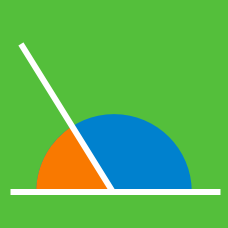Geometry

# Patterns In Geometry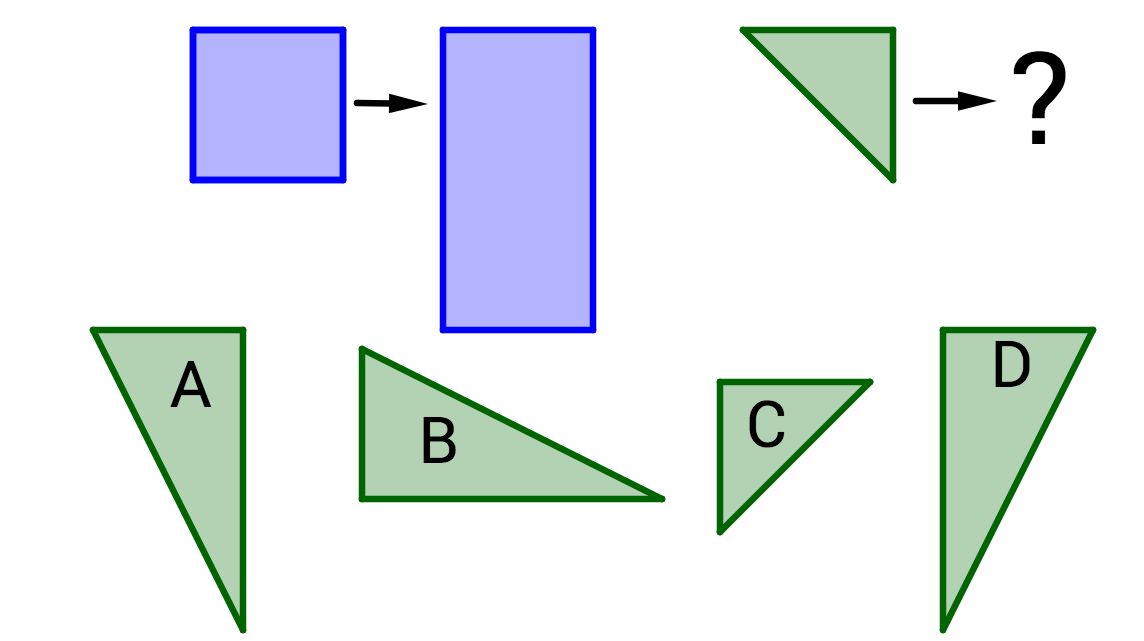The first blue rectangle is to the second blue rectangle as the green triangle is to $\text{\_\_\_\_\_\_\_}.$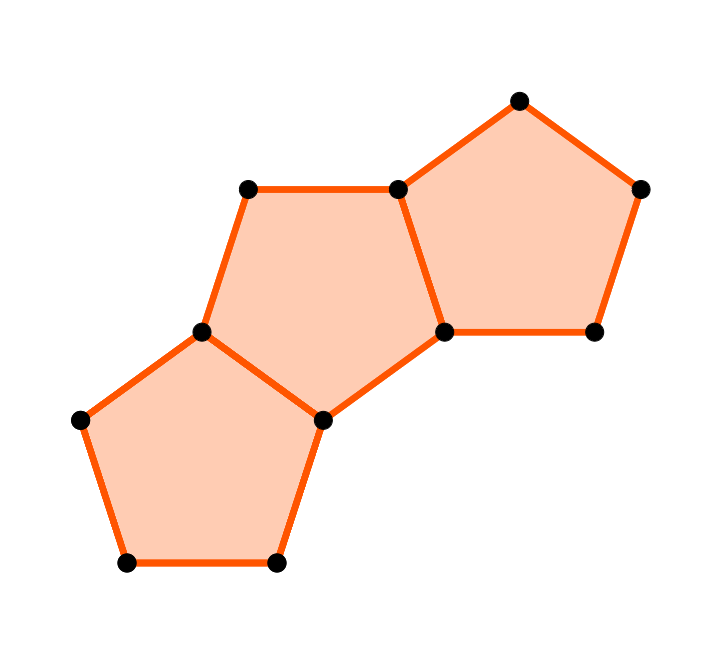Pentagon tables are placed end to end as shown to create one large table. One person can sit on each open side of a pentagon. For example, 5 people can sit at a pentagon table that is all by itself. How many people can at one large table created from five pentagons?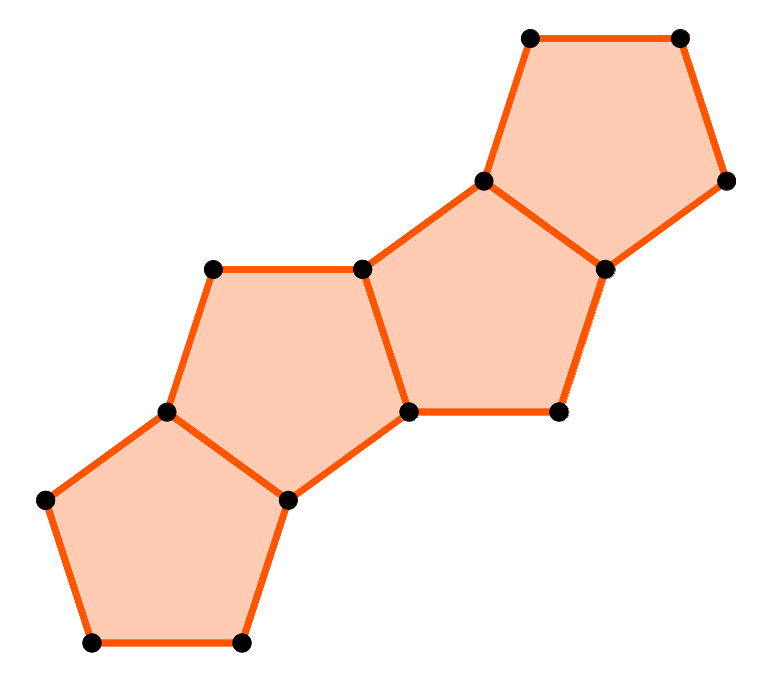If pentagon-shaped tables are placed end to end as shown to create one large table, and one person can sit on each open side of the table, how many tables are needed to seat 50 people?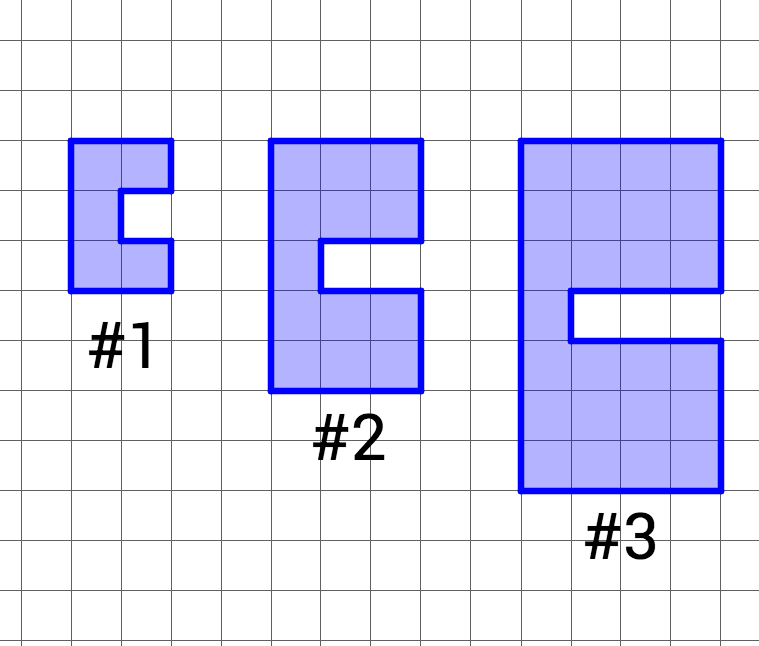How many blocks will be in Figure #10?Does Figure #5 have a height of 10 and a width of 6?

×